# Steady State Behavior of a Single-Switch Non-isolated DC-DC SEPIC Converter Topology with Improved Static Voltage Gain

Steady State Behavior of a Single-Switch Non-isolated DC-DC SEPIC Converter Topology with Improved Static Voltage Gain
Department of EEE, Government College of Engineering, Salem – 636 011, Tamil Nadu, India

Corresponding Author Email:
muralid36@yahoo.com
Page:
445-452
|
DOI:
https://doi.org/10.18280/jesa.540307
12 January 2021
|
Accepted:
17 May 2021
|
Published:
25 June 2021
| Citation

OPEN ACCESS

Abstract:

This paper presents the analysis of steady state behavior of a single switch non-isolated Single Ended Primary Inductance Converter (SEPIC) topology for achieving high DC voltage gain using diode-capacitor voltage multiplier. A voltage boosting module consisting of inductor and capacitor in addition with two diodes is introduced in the conventional SEPIC configuration in order to derive the DC-DC conversion technology proposed in this work. The voltage gain of the converter is extended using a diode-capacitor voltage multiplier cell. The converter suggested in this work has a single controlled switch. Hence, the conduction losses and the control complexity of the switch are very much reduced. The open loop configuration of the proposed non-isolated converter is described under continuous inductor current mode. The voltage boosting capability of the presented converter is compared with that of the existing modified SEPIC structure. The presented positive output converter topology has low switch voltage-current stress compared to the existing modified SEPIC topology given in the literature. The inductor and capacitor components of the suggested converter are so chosen that the DC output voltage and current waveforms show very low percentage of ripples. A DC voltage level of 24 V is given as input to the proposed converter. The DC voltage obtained across the load terminals is around 370 V which is achievable with low duty ratio (= 0.7) of the active switch. The voltage conversion ratio is very much influenced by the variation of the duty cycle of the power switch. In this work, the converter topology is presented and its various modes of operation are explained with equivalent circuits. The PSIM software platform is effectively and efficiently utilized to validate the performance of the converter. The obtained results convey that the proposed DC-DC conversion technology with extended voltage gain has the capability to maintain the steady-state output voltage and current profiles with almost negligible amount of ripples owing to the use of suitably designed non-dissipative elements in LC filter.

Keywords:

continuous inductor current mode, DC-DC conversion, diode-capacitor cell, duty ratio, extended voltage gain, PSIM, SEPIC topology, voltage boosting module

1. Introduction

During the past few years, both the population and industrial growth lead to increased electrical energy demand all over the world. The bulk amount of electrical energy can be generated using conventional sources (fossil fuels) such as coal, petroleum, and natural gas. But the method of generating electrical energy using non-renewable energy sources leads to pollution of surrounding environment due to carbon emissions from the electrical energy consumption and increased cost of the overall system. Moreover, the fossil fuels are depleting day by day. To protect the environment and reduce the global surface temperature, it is necessary to develop renewable form of energy sources. In the 1970s, the researchers began to concentrate on clean and sustainable energy sources such as photovoltaic (PV) panels, fuel cells, and wind power system [1, 2]. Among the various renewable energy sources available, solar resources have certain advantages such as reliability, environmental-friendly, low maintenance, and low operational cost. The DC output voltage generated by the solar cells is dependent on the weather conditions, and is of low value (around 15V – 40V) and is variable with respect to sunlight intensity [3, 4].

The low level of DC output voltage generated by PV panels should be converted to the high level of DC voltage in order to connect these PV panels to the grid or to the local consumers. Hence, it is required to have a power electronic interface between PV system and DC grid system . This interface can be a step-up DC-DC power electronic converter with extremely low duty ratio, which should be capable to produce high DC voltage from the low DC voltage generated by the PV panels.

There are many isolated and non-isolated DC-DC boost power electronic converter topologies in literature to achieve high DC output voltage [6-19]. The high voltage gain can be obtained using traditional single-switch DC-DC boost converter with increased value of duty cycle nearer to unity [20, 21]. But the duty cycle nearer to unity will increase the conduction and switching losses which might result in lower efficiency. There is another way of increasing the output voltage by connecting multiple boost converters in series. However, this scheme will increase the cost of the converter due to too many numbers of components. The isolated topologies use a high frequency transformer for isolating the DC input port and the DC output port. However, these isolated configurations can achieve high step-up voltage conversion ratio proportional to the size of the transformer. But the demerits of using transformer-based topologies are that (i). the leakage inductance of the transformer can cause voltage spikes on the active switches and high power dissipation, and (ii). electromagnetic interference (EMI) problems. Due to these limitations, the isolated topologies have reduced overall converter efficiency.

The high voltage gain can also be realized using coupled-inductor approach [22, 23]. But, in this method, the leakage inductance of the inductor causes high voltage spikes across the controlled power switches. An active clamp circuit can be used to resolve this issue . However, the transformer-less DC-DC converters can be implemented using switched-inductor and switched-capacitor approaches to achieve high DC output voltage with minimum value of duty ratio of the switches [25, 26]. These approaches also suffer from the drawbacks such as complexity, a greater number of components, increased input ripple current level, and poor voltage regulation. Moreover, high charging currents will flow through the main active switch thereby causing high conduction losses in the case of switched-capacitor structure. The voltage-lift structure based DC-DC converter used to obtain high voltage gain also suffers from the increased conduction losses due to high charging currents [27-29]. The above drawbacks are eliminated in interleaved boost topology . This topology can give high voltage conversion ratio when increased number of switches is employed in the circuit .

Because of higher efficiency and simple structure, non-isolated converter topologies are preferred. There are certain non-isolated single-switch DC-DC converter topologies such as Cuk, Zeta, conventional SEPIC, and modified SEPIC configurations for obtaining high static voltage gain [32, 33]. The modified SEPIC structure can give almost twice the static voltage gain as that produced by the conventional boost conversion technology . The voltage gain of the modified SEPIC topology can be extended using voltage multiplier cell (Diode-Capacitor cell) technique . This voltage multiplier based boost converters can be realized without any additional active switch. Moreover, the original configuration of the converter need not be modified because of the addition of Diode-Capacitor cell in the circuit . Many Diode-Capacitor cells can be added to extend the static gain of the converter. It is possible to step-up the DC output voltage level to higher value without using any magnetic elements in the cell.

The work proposed in this paper explains the continuous inductor current mode operation of a non-isolated single-switch positive output step-up DC-DC conversion technology. The proposed topology is derived from the conventional SEPIC structure in order to achieve high DC voltage gain. The high gain is obtained from the proposed configuration without using high duty ratio of the main switch. The switch is subjected to low voltage-current stress. Further, the DC output voltage level can be extended using diode-capacitor cell based voltage expander. The addition of diode-capacitor cell modifies the open-loop voltage gain of the converter in such a way that the DC output voltage of the converter with voltage expander is almost twice that of the converter  without voltage expander.

The remaining sections are planned as follows: Section 2 gives the detailed analysis of modes of operation followed by the steady state behavior of the proposed non-isolated step-up DC-DC conversion topology. The validation of the proposed converter configuration through PSIM simulation is presented in Section 3. Section 4 concludes the summary of the proposed research work.

2. Analysis of Circuit Configuration and Steady-State Behavior of the Proposed DC-DC Conversion Scheme

## 1.png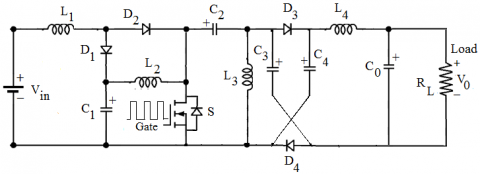Figure 1. Circuit configuration of the proposed single-switch controlled non-isolated positive output DC-DC boost converter

## 2.png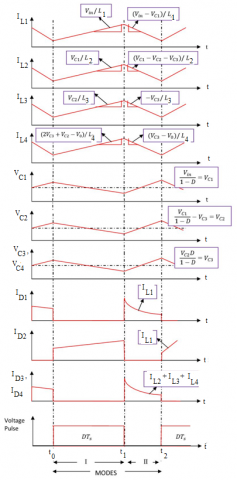Figure 2. Typical steady-state waveforms of the suggested converter during continuous inductor current mode

The topology of a single-switch non-isolated positive output boost converter capable of producing high DC output voltage is shown in Figure 1. The proposed topology is the extension of the converter configuration given in ref.  with voltage expander cell included. An input DC voltage of 24 V is given to the circuit. The DC output voltage appears across a load resistor RL. The proposed extended converter configuration employs three identical inductors L1, L2 and L3 with each of 1 mH inductance. There are two sets of identical capacitors: one set having C1 and C2 with each of 100 µF capacitance, another set having C3 and C4 with each of 10 µF capacitance. The large values of capacitances are chosen to achieve constant voltage. The power circuit employs four ideal diodes namely D1, D2, D3 and D4. The module formed by two diodes D3 and D4 and two capacitors D3 and D4 is called Diode-Capacitor voltage expander cell. The voltage gain of the converter proposed in  is extended using the Diode-Capacitor voltage multiplier module. The LC filter section consists of inductor L4 and capacitor C0. The filter components (L4=1 mH and C0=300 mF) are selected in such a way that the ripples are reduced in the output voltage and current. The ideal active high power semiconductor switch S is triggered into conduction by a proper gate pulse. All the inductors, capacitors, and diodes are controlled by the single switch S in the power circuit. In this work, the switching frequency (fs) of the switch is chosen as 50 kHz. The typical steady-state waveforms of the suggested converter configuration operating under continuous inductor current mode are presented in Figure 2. The circuit operation and the steady-state behavior of the converter under continuous inductor current mode are explained as shown in Figure 1 above.

2.1 Circuit operation of the converter during Mode-I

The high power semiconductor switch S of the converter under continuous inductor current conduction mode is switched on during the time interval t0-t1. This mode of operation of the converter is represented in Figure 3. The diode D2 gets forward-biased. The diodes D1, D3 and D4 get reverse-biased. The inductor L1 is magnetized with current flow path: Vin-VL1-D2-S-Vin. The inductor L2 gets magnetized from the capacitor C1 with current flow path: VC1-VL2-S-VC1. The inductor L3 gets magnetized from the capacitor C2 with current flow path: VC2-S-VL3-VC2. The inductor L4 gets magnetized from the capacitor C4 with current flow path: VC4-VL3-V0-VC3-VC4. The load resistor RL receives the energy from the capacitor C0. The following equations are obtained during Mode-I operation:

$\left\{ \begin{matrix} {{\text{V}}_{\text{in}}}={{\text{V}}_{\text{L}1}};{{\text{V}}_{\text{C}1}}={{\text{V}}_{\text{L}2}};{{\text{V}}_{\text{C}2}}={{\text{V}}_{\text{L}3}}; \\ 2{{\text{V}}_{\text{C}3}}+{{\text{V}}_{\text{C}2}}-{{\text{V}}_{0}}={{\text{V}}_{\text{L}4}};{{\text{V}}_{\text{C}4}}={{\text{V}}_{\text{C}3}} \\\end{matrix} \right.$              (1)

where, VL1, VL2, VL3 and VL4 represent the voltages across the inductors L1, L2, L3 and L4 respectively; VC1, VC2, VC3 and VC4 represent the voltages across the inductors C1, C2, C3 and C4 respectively.

## 3.png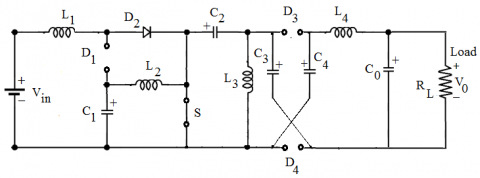Figure 3. Circuit representation of the converter during Mode-I operation

2.2 Circuit operation of the converter during Mode-II

The active switch S of the converter under continuous inductor current conduction mode is turned-off during the time interval t1-t2. This mode of operation of the converter is represented in Figure 4. The diode D2 gets biased in the reverse direction. The diodes D1, D3 and D4 get forward biased. The inductor L1 gets demagnetized through the path: Vin-VL1-D1-VC1-Vin. The inductor L2 gets demagnetized through the path: VC1-VL2-VC2-D3-VC4-VC1. The inductor L3 gets demagnetized through the path: VL3-VC3-D4-VL3. The inductor L4 gets demagnetized through the path: VC4-VL4-V0-D4-VC4. The voltages across the inductors are written as Eq. (2):

$\left\{ \begin{array}{*{35}{l}} {{V}_{in}}-{{V}_{Cl}}={{V}_{L1}};{{V}_{C1}}-{{V}_{C2}}-{{V}_{C4}}={{V}_{L2}} \\ -{{V}_{C3}}={{V}_{L3}};{{V}_{C3}}-{{V}_{0}}={{V}_{L4}};{{V}_{C4}}={{V}_{C3}} \\\end{array} \right.$                 (2)

By applying the volt-second balance principle for the inductors L1, L2, L3 and L4, the following Eqns. (3), (4), (5), and (6) can be obtained:

${{\text{V}}_{\text{C1}}}=\frac{{{\text{V}}_{\text{in}}}}{\text{(1-D)}}$                 (3)

$\frac{{{\text{V}}_{\text{C1}}}}{\text{(1-D)}}={{\text{V}}_{\text{C2}}}+{{\text{V}}_{\text{C3}}}$             (4)

$\text{D}{{\text{V}}_{\text{C2}}}=(1-\text{D)}{{\text{V}}_{\text{C3}}}$                 (5)

$(1+\text{D)}{{\text{V}}_{\text{C3}}}+\text{D}{{\text{V}}_{\text{C2}}}={{\text{V}}_{\text{0}}}$          (6)

where, D represents the duty ratio of the switch S.

By rearranging the Eqns. (3), (4), (5) and (6), the following voltage gain Eq. (7) is derived:

$\text{Voltage Gain}=\frac{{{\text{V}}_{\text{0}}}}{{{\text{V}}_{\text{in}}}}=\frac{2\text{D}}{{{\text{(1-D)}}^{\text{2}}}}$          (7)

The above Eq. (7) can be used to calculate output voltages (V0) for various input voltages (Vin) and duty ratios (D). The following Table 1 depicts the values of output voltages for various values of input voltages and duty ratios. For comparison of the analytical results, the input voltage range is considered as varying from 12 V to 48 V due to the fact that the DC voltage delivered by the input renewable energy source may be in the range from 12 V – 40 V approximately. In this work, the upper limit for the duty ratio (D) of the switch is chosen as 0.7 as D value above 0.7 leads to very high output voltages for the increased input voltages.

## 4.png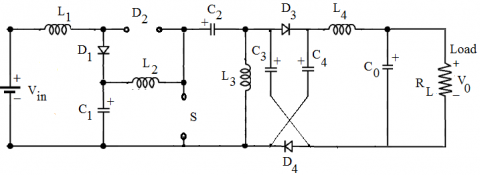Figure 4. Circuit representation of the converter during Mode-II operation

Table 1. Analytical results of the proposed converter

 Input voltage (Vin) V Output voltage (V0) V D = 0.4 D = 0.5 D = 0.6 D = 0.7 D = 0.8 12 26.67 48 90 186.72 480 24 53.33 96 180 373.44 960 36 80.00 144 270 560.16 1440 48 106.67 192 360 746.88 1920
3. PSIM Simulation Model of the Converter and the Results

Initially, the PSIM model of the non-isolated positive output DC-DC boost converter is developed without Diode-Capacitor (D-C) voltage multiplier cell as shown in Figure 5 and the converter operating under continuous inductor current mode is simulated in PSIM environment at a switching frequency of 50 kHz for the switch with duty ratio D = 0.7. The values of circuit components used for simulation are shown in Figure 5 itself. An input DC voltage of 24 V is given to the circuit as shown in Figure 9. The output DC voltage obtained is around 186 V and the output DC current is around 0.5 A for the converter without diode-capacitor cell. The waveforms of output voltage and output current are shown in Figure 6. Next, the PSIM model of the proposed converter is developed with a Diode-Capacitor voltage expander cell and an additional inductor L4 as shown in Figure 7. The parameters of the proposed converter are tuned in such a way that a high level of DC output voltage around 370 V is obtained across the load. Table 2 lists the values of parameters used for simulation of the suggested converter. The gate pulse given to the active switch S is indicated in Figure 8. The duty ratio ‘D’ of the switch is varied from 0.5 to 0.9 to validate the performance of the converter. However, at duty ratio D = 0.6 itself, high static voltage gain is obtained. The waveforms of DC input voltage, DC output voltage and DC output current are indicated in Figure 9, Figure 10, and Figure 11 respectively. The output DC voltage obtained is around 373 V and the output DC current is around 1 A for the proposed converter with diode-capacitor cell. The waveforms of voltages appearing across the four inductors are shown in Figure 12, Figure 13, Figure 14, and Figure 15 respectively. Figure 16 shows the current flow through the inductor L1. The remaining three inductors carry almost the same current as that of inductor L1. The charging and discharging voltage characteristics of the capacitors C1, C2, C3 and C4 are depicted in Figure 17, Figure 18, Figure 19, and Figure 20 respectively. The charging and discharging current waveforms of the four capacitors is shown in Figure 21. The voltage-current stress waveforms of the diodes D1, D2, D3 and D4 are depicted in Figure 22, Figure 23, Figure 24, Figure 25, and Figure 26 respectively. The voltage – current stresses across the active switch S are indicated in Figure 27 and Figure 28 respectively.

## 5.png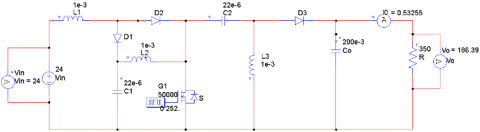Figure 5. PSIM model of the non-isolated DC-DC boost converter without Diode-Capacitor cell

## 6.png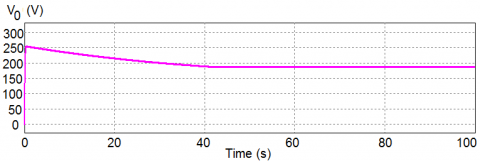(a)

## 6b.png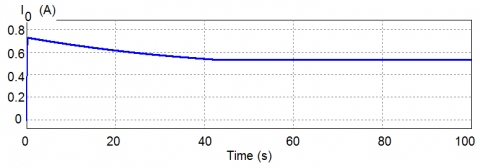(b)

Figure 6. (a) Waveforms of Output voltage (V0), and (b) Output current (I0) of the converter without Diode-Capacitor cell

## 7.pngFigure 7. PSIM model of the proposed non-isolated DC-DC boost converter with Diode-Capacitor cell

Table 2. Simulation parameters of the proposed converter

 Parameters Symbols Values Input voltage Vin 24 V (DC) Output voltage V0 370 V (DC) Inductors L1, L2, L3, L4 1 mH (each) Capacitors C1, C2 100 µF (each) Capacitors C3, C4 10 µF (each) Output capacitor C0 300 mF Switching frequency Fs 50 kHz Load resistance RL 350 Ω Output current I0 1 A Duty ratio of the switch S D 0.7

## 8.png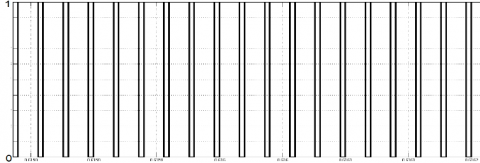Figure 8. Gating pulse to the switch S

## 9.png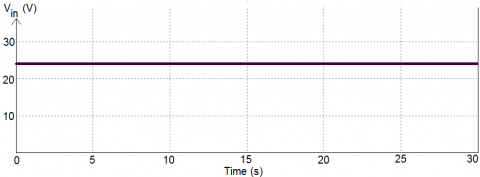Figure 9. Input voltage

## 10.png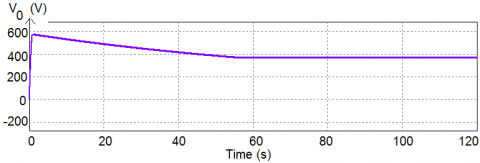Figure 10. Output voltage (V0)

## 11.png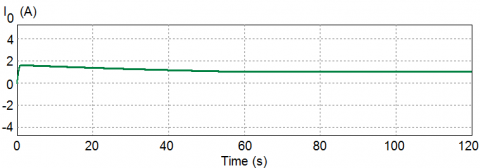Figure 11. Output current (I0)

## 12.png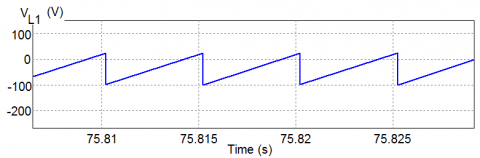Figure 12. Voltage across the inductor L1

## 13.png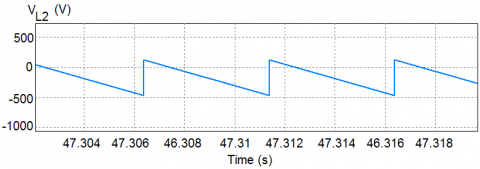Figure 13. Voltage across the inductor L2

## 14.png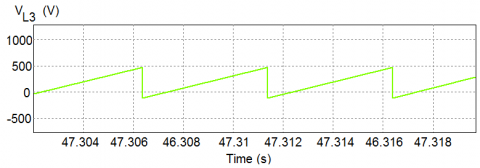Figure 14. Voltage across the inductor L3

## 15.png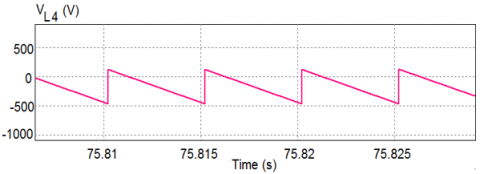Figure 15. Voltage across the inductor L4

## 16.png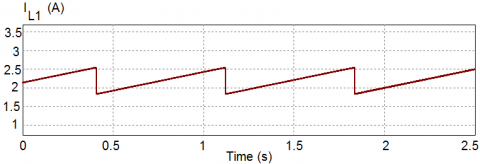Figure 16. Current through the inductor L1

## 17.pngFigure 17. Voltage across the capacitor C1

## 18.pngFigure 18. Voltage across the capacitor C2

## 19.png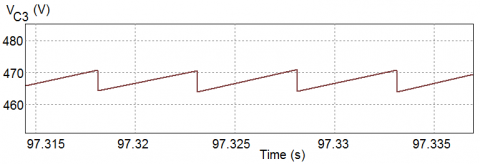Figure 19. Voltage across the capacitor C3

## 20.png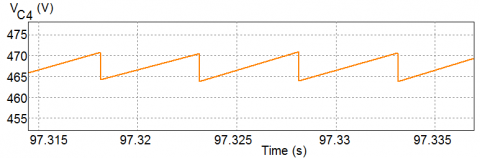Figure 20. Voltage across the capacitor C4

## 21.png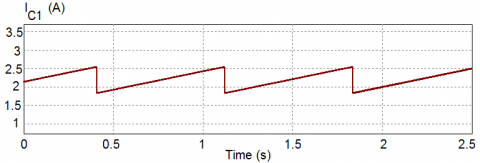Figure 21. Current through the capacitor C1

## 22.png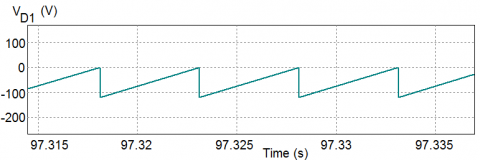Figure 22. Voltage across the Diode D1

## 23.png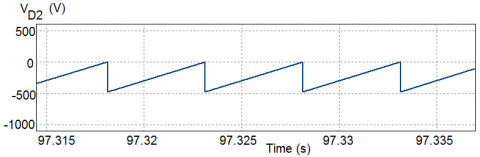Figure 23. Voltage across the Diode D2

## 24.png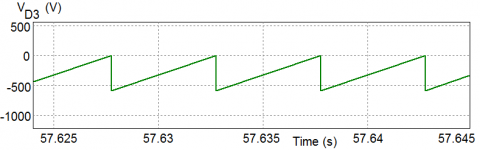Figure 24. Voltage across the Diode D3

## 25.png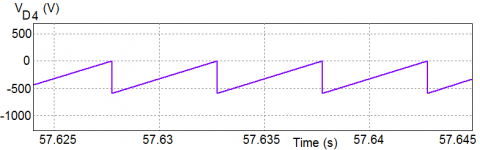Figure 25. Voltage across the Diode D4

## 26.png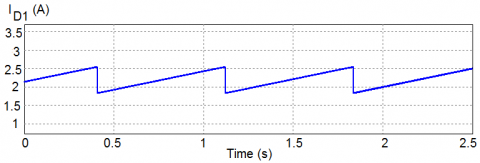Figure 26. Current through the Diodes

## 27.png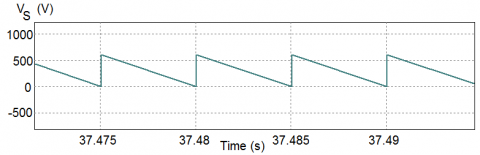Figure 27. Voltage across the Switch S

## 28.png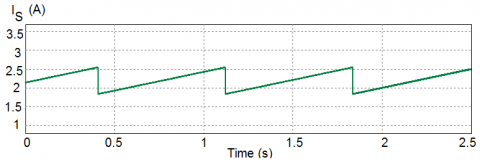Figure 28. Current through the Switch S

4. Conclusions

In this paper, the continuous inductor current mode analysis in terms of modes of operation and steady-state behavior of a non-isolated high step-up positive output DC-DC conversion scheme is presented in open-loop configuration with and without diode-capacitor voltage expander module. The PSIM simulation tool has been employed to validate the performance of the proposed converter topology. The results of simulation shown for duty ratio D = 0.7 demonstrate that the suggested converter configuration has the ability to realize a high level of DC output voltage which is almost fifteen times the DC input voltage. The improved DC output voltage profile is mainly due to the presence of diode-capacitor voltage multiplier cell. The waveforms of output voltage and output current are shown to have fewer amounts of ripples due to the proper selection of filter components. The static voltage gain of the converter has been derived in open-loop mode. The gain derived is twice that of the converter proposed in . The well-suited area of applications of the proposed converter is for high voltage renewable energy systems. The voltage and current waveforms of non-dissipative elements, diodes, and active switch are also analyzed for performance validation of the converter. The converter configuration suggested in this work employs a single MOSFET switch which is subjected to lower amounts of voltage and current stresses. In future work, the zero-voltage-switching concept may be employed for the switch in view of reduction of switching loss so that the converter efficiency may be improved.

Nomenclature
 D Duty ratio of the active switch L1, L2, L3, L4 C1, C2, C3, C4 C0 Inductances of inductors, mH Capcitances of capacitors, µF Filter capacitance, mF Vin Input DC voltage, V V0 Output DC voltage, V Subscripts in input o L output load
References

 Li, S., Haskew, T.A., Li, D., Hu, F. (2011). Integrating photovoltaic and power converter characteristics for energy extraction study of solar PV systems. Renewable Energy, 36(12): 3238-3245. https://doi.org/10.1016/j.renene.2011.02.016

 Zhao, Y., Xiang, X., Li, C., Gu, Y., Li, W., He, X. (2014). Single-phase high step-up converter with improved multiplier cell suitable for half-bridge-based PV inverter system. IEEE Transactions on Power Electronics, 29(6): 2807-2816. https://doi.org/10.1109/TPEL.2013.2273975

 Hossain, M.Z., Rahim, N.A., Selvaraj, J. (2018). Recent progress and development on power DC-DC converter topology, control, design and applications: A review. Renewable and Sustainable Energy Reviews, 81(1): 205-230. https://doi.org/10.1016/j.rser.2017.07.017

 El-Helw, H.M., Al-Hasheem, M., Marei, M.I. (2016). Control strategies for the DAB based PV interface system. PLOS ONE, 11(8): 1-19. https://doi.org/10.1371/journal.pone.0161856

 Jayaraju, G., Rao, G.S. (2019). A new optimized ANN algorithm based single phase grid connected PV-Wind system using single switch high gain DC-DC converter. European Journal of Electrical Engineering, 21(4): 373-381. https://doi.org/10.18280/ejee.210405

 Kenzelmann, S., Rufer, A., Dujic, D., Canales, F., de Novaes, Y.R. (2015). Isolated dc/dc structure based on modular multilevel converter. IEEE Transactions on Power Electronics, 30(1): 89-98. https://doi.org/10.1109/TPEL.2014.2305976.

 He, P., Khaligh, A. (2017). Comprehensive analyses and comparison of 1 kW isolated DC-DC converters for bidirectional EV charging systems. IEEE Transactions on Transportation Electrification, 3(1): 147-156. https://doi.org/10.1109/TTE.2016.2630927

 Chub, A., Vinnikov, D., Blaabjerg, F., Peng, F.Z. (2016). A review of galvanically isolated impedance-source DC-DC converters. IEEE Transactions on Power Electronics, 31(4): 2808-2828. https://doi.org/10.1109/TPEL.2015.2453128

 Annapurani, S., Murali, D. (2020). Parallel-charge series-discharge inductor-based voltage boosting technique applied to a rectifier-fed positive output DC-DC converter. Journal of Applied Research and Technology, 18(5): 229-244. https://doi.org/10.22201/icat.24486736e.2020.18.5.1279

 Behera, R., Sahoo, S.K., Balamurugan, M., Shanmugam, P.K., Rani. C. (2020). PV based high gain boost converter. Soft Computing for Problem Solving, Advances in Intelligent Systems and Computing, 1048: 563-572. https://doi.org/10.1007/978-981-15-0035-0_46

 Shahir, F.M., Babaei, E., Farsadi, M. (2019). Extended topology for a boost dc-dc converter. IEEE Transactions on Power Electronics, 34(3): 2375-2384. https://doi.org/10.1109/TPEL.2018.2840683

 Wu, G., Ruan, X., Ye, Z. (2018). High step-up dc-dc converter based on switched-capacitor and coupled inductor. IEEE Transactions on Industrial Electronics, 65(7): 5572-5579. https://doi.org/10.1109/TIE.2017.2774773

 Shahir, F.M., Babaei, E., Farsadi, M. (2017). A new structure for non-isolated boost dc-dc converter. Journal of Circuits, Systems, and Computers, 26(1): 1-26. https://doi.org/10.1142/S0218126617500128

 Forouzesh, M., Shen, Y., Yari, K., Siwakoti, Y.P., Blaabjerg, F. (2018). High-efficiency high step-up dc-dc converter with dual coupled inductors for grid-connected photovoltaic systems. IEEE Transactions on Power Electronics, 33(7): 5967-5982. https://doi.org/10.1109/TPEL.2017.2746750

 Tseng, K.C., Cheng, C.A., Chen, C.T. (2017). High step-up interleaved boost converter for distributed generation using renewable and alternative power sources. IEEE Journal of Emerging and Selected Topics in Power Electronics, 5(2): 713-722. https://doi.org/10.1109/JESTPE.2016.2611641

 Dobi, A.H.M., Sahid, M.R. (2020). Non-isolated LLC resonant DC-DC converter with balanced rectifying current and stress. Indonesian Journal of Electrical Engineering and Computer Science, 18(2): 707-716. https://doi.org/10.11591/ijeecs.v18.i2.pp698-706

 Saravanan, S., Ramesh Babu, N. (2019). A modified high step-up non-isolated DC-DC converter for PV application. Journal of Applied Research and Technology, 15(3): 242-249. https://doi.org/10.22201/icat.16656423.0.15.3.655

 Murali, D., Annapurani, S. (2019). A high voltage gain rectifier-fed two-stage step-up DC-DC converter with PI controller. International Journal of Engineering Applied Sciences and Technology, 4(8): 160-164. https://doi.org/10.33564/IJEAST.2019.v04i08.024

 Sowmya, A., Murali, D. (2019). A high voltage gain step-up resonant DC-DC converter topology with reduced switch count. International Journal for Modern Trends in Science and Technology, 5(12): 26-31. https://doi.org/10.46501/IJMTST051205

 Hsieh, Y.P., Chen, J.F., Liang, T.J., Yang, L.S. (2013). Novel high step-up DC-DC converter for distributed generation system. IEEE Transactions on Industrial Electronics, 60(4): 1473-1482. https://doi.org/10.1109/TIE.2011.2107721

 Hsieh, Y.P., Chen, J.F., Liang, T.J., Yang, L.S. (2012). Novel high step-up DC-DC converter with coupled-inductor and switched-capacitor techniques. IEEE Transactions on Industrial Electronics, 59(2): 998-1007. https://doi.org/10.1109/TIE.2011.2151828

 Hu, X., Gong, C. (2014). A high voltage gain DC-DC converter integrating coupled-inductor and diode-capacitor techniques. IEEE Transactions on Power Electronics, 29(2): 789-800. https://doi.org/10.1109/TPEL.2013.2257870

 Gao, Y., Liu, H., Ai, J. (2018). Novel high step-up DC-DC converter with three-winding-coupled-inductors and its derivatives for a distributed generation system. Energies Journal, 11(12): 1-12. https://doi.org/10.3390/en11123428

 Liu, H., Li, F. (2016). A novel high step-up converter with a quasi-active switched-inductor structure for renewable energy systems. IEEE Transactions on Power Electronics, 31(7): 5030-5039. https://doi.org/10.1109/TPEL.2015.2480115

 Hsieh, Y.P., Chen, J.F., Liang, T.J., Yang, L.S. (2011). Novel high step-up DC-DC converter with coupled-inductor and switched-capacitor techniques for a sustainable energy system. IEEE Transactions on Power Electronics, 26(12): 3481-3490. https://doi.org/10.1109/TPEL.2011.2160876

 Kim, H.Y., Moon, E.A., Nguyen, M.K. (2019). A novel negative-output high step-up ratio DC-DC converter based on switched-inductor cell. Journal of Korean Electrical and Electronics Engineers, 23(1): 273-279. https://doi.org/10.7471/ikeee.2019.23.1.273

 Liang, T.J., Lee, J.H., Chen, S.M., Chen, J.F., Yang, L.S. (2013). Novel isolated high-step-up DC-DC converter with voltage lift. IEEE Transactions on Industrial Electronics, 60(4): 1483-1491. https://doi.org/10.1109/TIE.2011.2177789

 Liu, H.C., Li, F. (2015). Novel high step-up DC-DC converter with active coupled-inductor network for a sustainable energy system. IEEE Transactions on Power Electronics, 30(12): 6476-6482. https://doi.org/10.1109/TPEL.2015.2429651

 Adlakha, R., Khosla, A., Joshi, D. (2020). Comparative analysis of positive output super lift DC-DC luo converters. Indonesian Journal of Electrical Engineering and Computer Science, 18(2): 707-716. https://doi.org/10.11591/ijeecs.v18.i2.pp707-716

 Latha Shenoy, K., Gurudas Nayak, C., Mandi, R.P. (2017). Design and implementation of interleaved boost converter. International Journal of Engineering and Technology, 9(3S): 496-502. https://doi.org/10.21817/ijet/2017/v9i3/170903S076

 Hyuntae, C., Minsoo, J., Mihai, C., Agelidis, G. (2016). Performance evaluation of interleaved high- gain converter configurations. IET Power Electronics, 9(9): 1852-1861. https://doi.org/10.1049/iet-pel.2015.0644

 Siddharthan, N., Balasubramanian, B. (2019). Performance evaluation of SEPIC, Luo and ZETA converter. International Journal of Power Electronics and Drive Systems, 10(1): 374-380. https://doi.org/10.11591/ijpeds.v10.i1.pp374-380

 Maroti, P.K., Padmanaban, S., Nielsen, J.B.H., Bhaskar, M.S., Meraj, M., Iqbal, A. (2019). A new structure of high voltage gain SEPIC converter for renewable energy applications. IEEE Access, 7: 89857-89868. https://doi.org/10.1109/ACCESS.2019.2925564

 De Melo, P.F., Gules, R., Romaneli, E.F.R., Annunziato, R.C. (2010). A modified SEPIC converter for high-power-factor rectifier and universal input voltage applications. IEEE Transactions on Power Electronics, 25(2): 310-321. https://doi.org.10.1109/TPEL.2009.2027323

 Divya Navamani, J., Lavanya, A., Vijayakumar, K. (2019). Modified SEPIC converter with high boosting capability. Electronics Letters, 55(13): 759-761. https://doi.org/10.1049/el.2019.0836

 Divya Navamani, J., Vijayakumar, K., Jegatheesan, R. (2018). Non-isolated high gain DC-DC converter by quadratic boost converter and voltage multiplier cell. Ain Shams Engineering Journal (Electrical Engineering), 9(4): 1397-1406. https://doi.org/10.1016/j.asej.2016.09.007